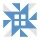Home Communities
IT Knowledge
Inspiration
Languages
EN

# JavaScript - calculate cos in degrees

3 points
Created by:May87
727

In this article, we would like to show you a simple example of how to calculate cos in degrees in JavaScript.

In order to use this example we just need to change the value of `deg` variable to some other value (in degrees).

``````// ONLINE-RUNNER:browser;

function calculateCos(deg) {
var rad = (Math.PI / 180) * deg;

}

var deg = 30;
var y = calculateCos(deg);

// cos(30 deg) = 0.8660254037844387
console.log('cos(' + deg + ' deg) = ' + y);``````

We can change `deg` to other values.

Example of common calculations:

``````cos(0 deg) = 1
cos(30 deg) = 0.8660254037844387
cos(45 deg) = 0.7071067811865476
cos(60 deg) = 0.5000000000000001
cos(90 deg) = 6.123233995736766e-17``````

I've prepared a similar post but for calculation sin in degrees:

## Alternative titles

Join to our subscribers to be up to date with content, news and offers.